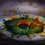# Recurrences using Programming !!!!

It's possible to generalize many of the recurrence relations (that we come across in Combinatorics problems, (you may want to try recurrence relations problems like these )

To learn how to generalize recurrence relations (using math) you could read these notes by Daniel Chiu - recursion, linear, part 2.

And if you want to know some tricks to manipulate things come to homogeneous recurrences, see the solutions in my note Need help

This was for in Maths... Let's now try programming..... (I have just started learning it...)

1. The famous Fibonacci sequence.... It is defined as $a_0=0$, $a_1=1$ and for $n\geq 2$ $a_n = a_{n-1} + a_{n-2}$

In programming(Python), people try various programs to print the numbers, but what i prefer is , start with an empty list "$\color{#D61F06}{\textbf{ li=[ ] }}$ "

Then, we write a program which will take into consideration all the Fibonacci numbers.recurnce

As shown in the image, just $\color{#D61F06}{\textbf{append}}$ the $b$ to $\color{#D61F06}{\textbf{li}}$ , so the $\color{#D61F06}{\textbf{list "li"}}$ is now containing the terms of Fibonacci Sequence!!!

On this list, as shown you can do operations like $sum$ of elements (use sum(li) ), $number$ of elements (use len(li), which gives length of list 'li'), and the $li.count$ code can be used to see whether any given number is a term of a Fibonacci sequence or not. (If the number is in Fibonacci sequence, then $\color{#D61F06}{\text{ li.count(number)}}$ will give value 1, and else, 0 )

This will be for the terms of Fibonacci sequence which are less than the range you type at first. (in the while loop)

Also, not only Fibonacci sequence, you can get other recurrence relations by this too !

Like the one in $3$ terms , like initial conditions $a_0=0,a_1=2,a_2=4$ and for $n\geq 3$, $a_n=7a_{n-1}+8a_{n-2}-4a_{n-3}$ This type of recurrences, you can solve by making characteristic equation and finding it's roots, as done in this note.

But by programming, it will be as followsrecrnc

In this one, the recurrence is simply defined by the last line in the loop. Then, the list will directly get numbers included and you can try any operations, like sum of first $n$ terms, or you can even find the $n^{th}$ term of the sequence just by using python code $\color{#D61F06}{\text{ li [n : (n+1) ]}}$ and it will give you the $n^{th}$ term. (Note that it won't give general form, it will give value for value of $n$ ).

Because this was example, i restricted the range of $c$ , but you may try to get bigger values by increasing it !

This shows the use of Programming to get terms of a recurrence relation and easily doing operations on them, like addition of few, for example, if you want the sum of terms $F_{3}$ to $F_7$ of Fibonacci sequence (i.e. $\displaystyle \sum_{n=3}^7 F_n$ , where $F_n$ is Finbonacci $n^{th}$ term), then you define a new list as $\color{#D61F06}{\textbf{li\_1 = li[2:7] }}$ and it will make $\color{#D61F06}{\textbf{li\_1}}$ to be list of Fibonacci numbers from $F_3$ to $F_7$. (Note that in new list, we took $2$ in formula for li_1 because it will exclude 2nd term)

Like and reshare if it was helpful to you.6 years, 12 months ago

This discussion board is a place to discuss our Daily Challenges and the math and science related to those challenges. Explanations are more than just a solution — they should explain the steps and thinking strategies that you used to obtain the solution. Comments should further the discussion of math and science.

When posting on Brilliant:

• Use the emojis to react to an explanation, whether you're congratulating a job well done , or just really confused .
• Ask specific questions about the challenge or the steps in somebody's explanation. Well-posed questions can add a lot to the discussion, but posting "I don't understand!" doesn't help anyone.
• Try to contribute something new to the discussion, whether it is an extension, generalization or other idea related to the challenge.

MarkdownAppears as
*italics* or _italics_ italics
**bold** or __bold__ bold
- bulleted- list
• bulleted
• list
1. numbered2. list
1. numbered
2. list
Note: you must add a full line of space before and after lists for them to show up correctly
paragraph 1paragraph 2

paragraph 1

paragraph 2

[example link](https://brilliant.org)example link
> This is a quote
This is a quote
    # I indented these lines
# 4 spaces, and now they show
# up as a code block.

print "hello world"
# I indented these lines
# 4 spaces, and now they show
# up as a code block.

print "hello world"
MathAppears as
Remember to wrap math in $$ ... $$ or $ ... $ to ensure proper formatting.
2 \times 3 $2 \times 3$
2^{34} $2^{34}$
a_{i-1} $a_{i-1}$
\frac{2}{3} $\frac{2}{3}$
\sqrt{2} $\sqrt{2}$
\sum_{i=1}^3 $\sum_{i=1}^3$
\sin \theta $\sin \theta$
\boxed{123} $\boxed{123}$

Sort by:

Very good discovery. This is fundamental to programming theory.

- 6 years, 12 months ago

??? trolling or what ? I know you already know this all....

- 6 years, 12 months ago

The notes were by Daniel Chiu, not me. I fixed it for you ;)

- 6 years, 12 months ago

Oops LOL

- 6 years, 11 months ago